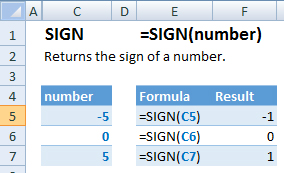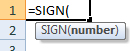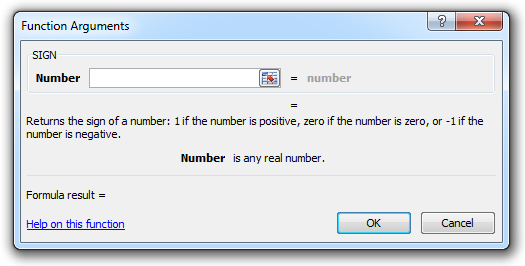### SIGN Function Examples – Excel & Google Sheets

This Excel Tutorial demonstrates how to use the Excel SIGN Function in Excel to calculate the sign, with formula examples.

## SIGN Function Description:

The SIGN Function Returns the sign of a number. 1 if positive. -1 if negative. 0 if zero.

## Formula Examples:Example Formula Result
1 =SIGN(C5) -1
2 =SIGN(C6) 0
3 =SIGN(C7) 1
AutoMacro - VBA Code Generator

## Syntax and Arguments:

The Syntax for the SIGN Formula is:

`=SIGN(number)`

### Function Arguments ( Inputs ):

number – A number.

## Additional Notes

Use the SIGN Function to calculate the sign of an angle. Simply enter the angle directly into the function or reference a cell containing the angle.

Return to the List of all Functions in Excel

## How to use the SIGN Function in Excel:

To use the AND Excel Worksheet Function, type the following into a cell:
`=AND(`
After entering it in the cell, notice how the AND formula inputs appear below the cell:You will need to enter these inputs into the function. The function inputs are covered in more detail in the next section. However, if you ever need more help with the function, after typing “=SIGN(” into a cell, without leaving the cell, use the shortcut CTRL + A (A for Arguments) to open the “Insert Function Dialog Box” for detailed instructions:For more information about the SIGN Formula visit the
Microsoft Website.

## Excel Practice Worksheet

Practice Excel functions and formulas with our 100% free practice worksheets!

• Automatically Graded Exercises
• Learn Excel, Inside Excel!